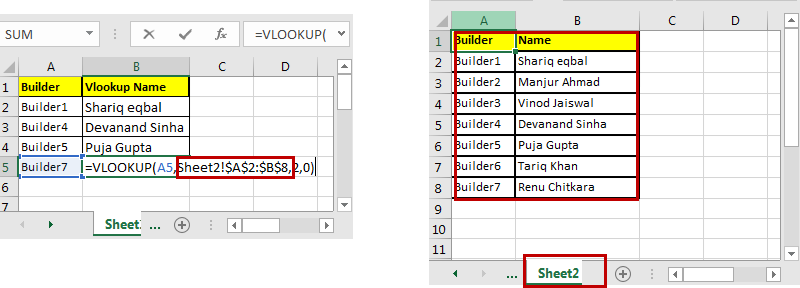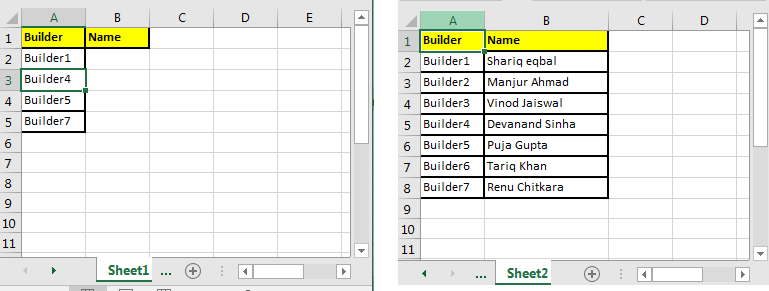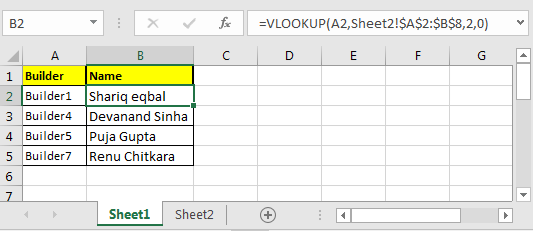# How to VLOOKUP from Different Excel Sheet in Excel

Using VLOOKUP to retrieve data from a different sheet is easier than you think.Generic Formula of VLOOKUP from Different Sheet

=VLOOKUP(lookup_value, sheetname!table_array, col_index,1/0)

I have explained how to use the VLOOKUP Function in Excel already. If you don’t know the functioning of VLOOKUP formula in Excel, I suggest you to check it out.

Here, the only differentiating factor is sheet name! from a normal VLOOKUP function. Just write the correct sheet name before table_array with "!" mark. Excel will VLOOKUP from that given sheet. Let's have a simple example.

VLOOKUP Example
On sheet1, I have Builder id. On sheet2, I have the name of those builders. So I just need to fetch the names from sheet2 to sheet1 using VLOOKUP.Write this formula in cell B2 on sheet1.

=VLOOKUP(A2,Sheet2!\$A\$2:\$B\$8,2,0)

Note the sheet2 here. If you rename it to sheet3, VLOOKUP will look for data on sheet 3 at a range \$A\$2:\$B\$8 (locked the reference to range) if it exists otherwise #REF error will be shown.Is that it? Yup, what you thought? Told you it is easier than you think. Let me know if this was useful to you. Or if you were looking for something else, let me know in the comment section below.

Related Articles:

How to Get Sheet name only in Excel

How to Select From Drop Down And Pull Data From Different Sheet in Microsoft Excel

How to Sum across sheets in Excel

How to use the SHEETS Function in Excel

How to Work With Named Ranges on Different Sheets in Excel

How To Loop Through Sheets In Excel Using VBA

Popular Articles:

50 Excel Shortcuts to Increase Your Productivity

How to use the VLOOKUP Function in Excel

How to use the COUNTIF function in Excel

How to use the SUMIF Function in Excel

Terms and Conditions of use

The applications/code on this site are distributed as is and without warranties or liability. In no event shall the owner of the copyrights, or the authors of the applications/code be liable for any loss of profit, any problems or any damage resulting from the use or evaluation of the applications/code.